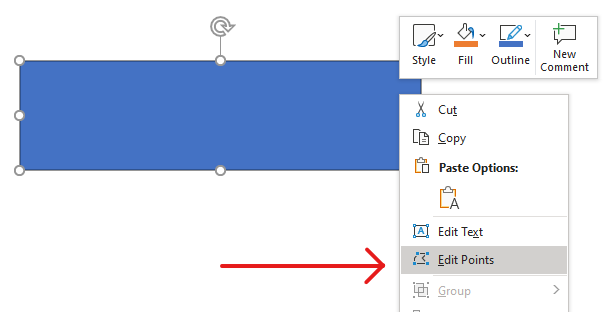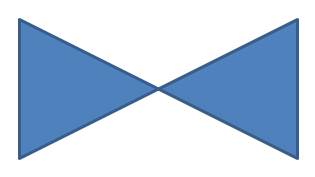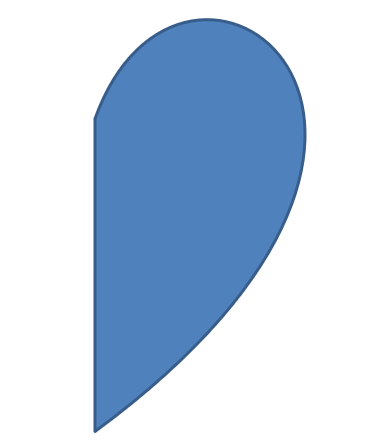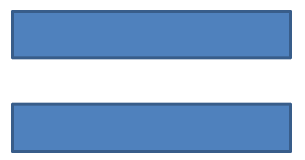Contents
[ ]

# Change a Shape Using Edit Points

Consider a square. In PowerPoint, using edit points, you can

• move the square’s corner in or out
• specify the curvature for a corner or point
• add new points to the square
• manipulate points on the square, etc.

Essentially, you can perform the described tasks on any shape. Using edit points, you get to change a shape or create a new shape from an existing shape.

## Shape Editing TipsBefore you start editing PowerPoint shapes through edit points, you might want to consider these points about shapes:

• A shape (or its path) can either be closed or open.
• When a shape is closed, it lacks a start or end point. When a shape is open, it has a beginning and end.
• All shapes consist of at least 2 anchor points linked to each other by lines
• A line is either straight or curved. Anchor points determine the nature of the line.
• Anchor points exist as corner points, straight points, or smooth points:
• A corner point is a point where 2 straight lines join at an angle.
• A smooth point is a point where 2 handles exist in a straight line and the line’s segments join in a smooth curve. In this case, all handles are separated from the anchor point by an equal distance.
• A straight point is a point where 2 handles exist in a straight line and that line’s line segments joins in a smooth curve. In this case, the handles don’t have to be separated from the anchor point by an equal distance.
• By moving or editing anchor points (which changes the angle of lines), you can change the way a shape looks.

To edit PowerPoint shapes through edit points, Aspose.Slides provides the GeometryPath class and IGeometryPath interface.

## Simple Editing Operations

This python code shows you how to

Add a line to the end of a path:

``````line_to(point)
line_to(x, y)
``````

Add a line to a specified position on a path:

``````line_to(point, index)
line_to(x, y, index)
``````

Add a cubic Bezier curve at the end of a path:

``````cubic_bezier_to(point1, point2, point3)
cubic_bezier_to(x1, y1, x2, y2, x3, y3)
``````

Add a cubic Bezier curve to the specified position on a path:

``````cubic_bezier_to(point1, point2, point3, index)
cubic_bezier_to(x1, y1, x2, y2, x3, y3, index)
``````

``````quadratic_bezier_to(point1, point2)
``````

``````quadratic_bezier_to(point1, point2, index)
``````

Append a given arc to a path:

``````arc_to(width, heigth, startAngle, sweepAngle)
``````

Close the current figure of a path:

``````close_figure()
``````

Set the position for the next point:

``````move_to(point)
move_to(x, y)
``````

Remove the path segment at a given index:

``````remove_at(index)
``````

## Add Custom Points to Shape

1. Create an instance of the GeometryShape class and set the ShapeType.Rectangle
2. Get an instance of the GeometryPath class from the shape.
3. Add a new point between the two top points on the path.
4. Add a new point between the two bottom points on the path.
5. Apply the path to the shape.

This python code shows you how to add custom points to a shape:

``````import aspose.slides as slides

with slides.Presentation() as pres:
shape = pres.slides.shapes.add_auto_shape(slides.ShapeType.RECTANGLE, 100, 100, 200, 100)
geometryPath = shape.get_geometry_paths()

geometryPath.line_to(100, 50, 1)
geometryPath.line_to(100, 50, 4)
shape.set_geometry_path(geometryPath)
``````## Remove Points from Shape

1. Create an instance of the GeometryShape class of and set the ShapeType.Heart type.
2. Get an instance of the GeometryPath class from the shape.
3. Remove the segment for the path.
4. Apply the path to the shape.

This python code shows you how to remove points from a shape:

``````import aspose.slides as slides

with slides.Presentation() as pres:
shape = pres.slides.shapes.add_auto_shape(slides.ShapeType.HEART, 100, 100, 300, 300)

path = shape.get_geometry_paths()
path.remove_at(2)
shape.set_geometry_path(path)
``````## Create Custom Shape

1. Calculate points for the shape.
2. Create an instance of the GeometryPath class.
3. Fill the path with the points.
4. Create an instance of the GeometryShape class.
5. Apply the path to the shape.

This python code shows you how to create a custom shape:

``````import aspose.slides as slides
import aspose.pydrawing as draw
import math

points = []

R = 100
r = 50
step = 72

for angle in range(-90, 270, step):
radians = angle * (math.pi / 180)
points.append(draw.PointF(x + R, y + R))

radians = math.pi * (angle + step / 2) / 180.0
points.append(draw.PointF(x + R, y + R))

starPath = slides.GeometryPath()
starPath.move_to(points)

for i in range(len(points)):
starPath.line_to(points[i])

starPath.close_figure()

with slides.Presentation() as pres:
shape = pres.slides.shapes.add_auto_shape(slides.ShapeType.RECTANGLE, 100, 100, R * 2, R * 2)
shape.set_geometry_path(starPath)
``````## Create Composite Custom Shape

1. Create an instance of the GeometryShape class.
2. Create a first instance of the GeometryPath class.
3. Create a second instance of the GeometryPath class.
4. Apply the paths to the shape.

This python code shows you how to create a composite custom shape:

``````import aspose.slides as slides
import aspose.pydrawing as draw

with slides.Presentation() as pres:
shape = pres.slides.shapes.add_auto_shape(slides.ShapeType.RECTANGLE, 100, 100, 200, 100)

geometryPath0 = slides.GeometryPath()
geometryPath0.move_to(0, 0)
geometryPath0.line_to(shape.width, 0)
geometryPath0.line_to(shape.width, shape.height/3)
geometryPath0.line_to(0, shape.height / 3)
geometryPath0.close_figure()

geometryPath1 = slides.GeometryPath()
geometryPath1.move_to(0, shape.height/3 * 2)
geometryPath1.line_to(shape.width, shape.height / 3 * 2)
geometryPath1.line_to(shape.width, shape.height)
geometryPath1.line_to(0, shape.height)
geometryPath1.close_figure()

shape.set_geometry_paths([ geometryPath0, geometryPath1])
``````## Create Custom Shape With Curved Corners

This python code shows you how to create a custom shape with curved corners (inwards):

``````import aspose.slides as slides
import aspose.pydrawing as draw

shapeX = 20
shapeY = 20
shapeWidth = 300
shapeHeight = 200

leftTopSize = 50
rightTopSize = 20
rightBottomSize = 40
leftBottomSize = 10

with slides.Presentation() as presentation:
slides.ShapeType.CUSTOM, shapeX, shapeY, shapeWidth, shapeHeight)

geometryPath = slides.GeometryPath()

point1 = draw.PointF(leftTopSize, 0)
point2 = draw.PointF(shapeWidth - rightTopSize, 0)
point3 = draw.PointF(shapeWidth, shapeHeight - rightBottomSize)
point4 = draw.PointF(leftBottomSize, shapeHeight)
point5 = draw.PointF(0, leftTopSize)

geometryPath.move_to(point1)
geometryPath.line_to(point2)
geometryPath.arc_to(rightTopSize, rightTopSize, 180, -90)
geometryPath.line_to(point3)
geometryPath.arc_to(rightBottomSize, rightBottomSize, -90, -90)
geometryPath.line_to(point4)
geometryPath.arc_to(leftBottomSize, leftBottomSize, 0, -90)
geometryPath.line_to(point5)
geometryPath.arc_to(leftTopSize, leftTopSize, 90, -90)

geometryPath.close_figure()

childShape.set_geometry_path(geometryPath)

presentation.save("output.pptx", slides.export.SaveFormat.PPTX)
``````

## Conversion of GeometryPath to GraphicsPath (System.Drawing.Drawing2D)

1. Create an instance of the GeometryShape class.
2. Create an instance of the GrpahicsPath class of the System.Drawing.Drawing2D namespace.
3. Convert the GraphicsPath instance to the GeometryPath instance using ShapeUtil.
4. Apply the paths to the shape.

This python code—an implementation of the steps above—demonstrates the GeometryPath to GraphicsPath conversion process:

``````import aspose.slides as slides
import aspose.pydrawing as draw

with slides.Presentation() as pres:
shape = pres.slides.shapes.add_auto_shape(slides.ShapeType.RECTANGLE, 100, 100, 300, 100)

originalPath = shape.get_geometry_paths()
originalPath.fill_mode = slides.PathFillModeType.NONE

gPath = draw.drawing2d.GraphicsPath()

gPath.add_string("Text in shape", draw.FontFamily("Arial"), 1, 40, draw.PointF(10, 10), draw.StringFormat.generic_default)

textPath = slides.util.ShapeUtil.graphics_path_to_geometry_path(gPath)
textPath.fill_mode = slides.PathFillModeType.NORMAL

shape.set_geometry_paths([originalPath, textPath])
``````